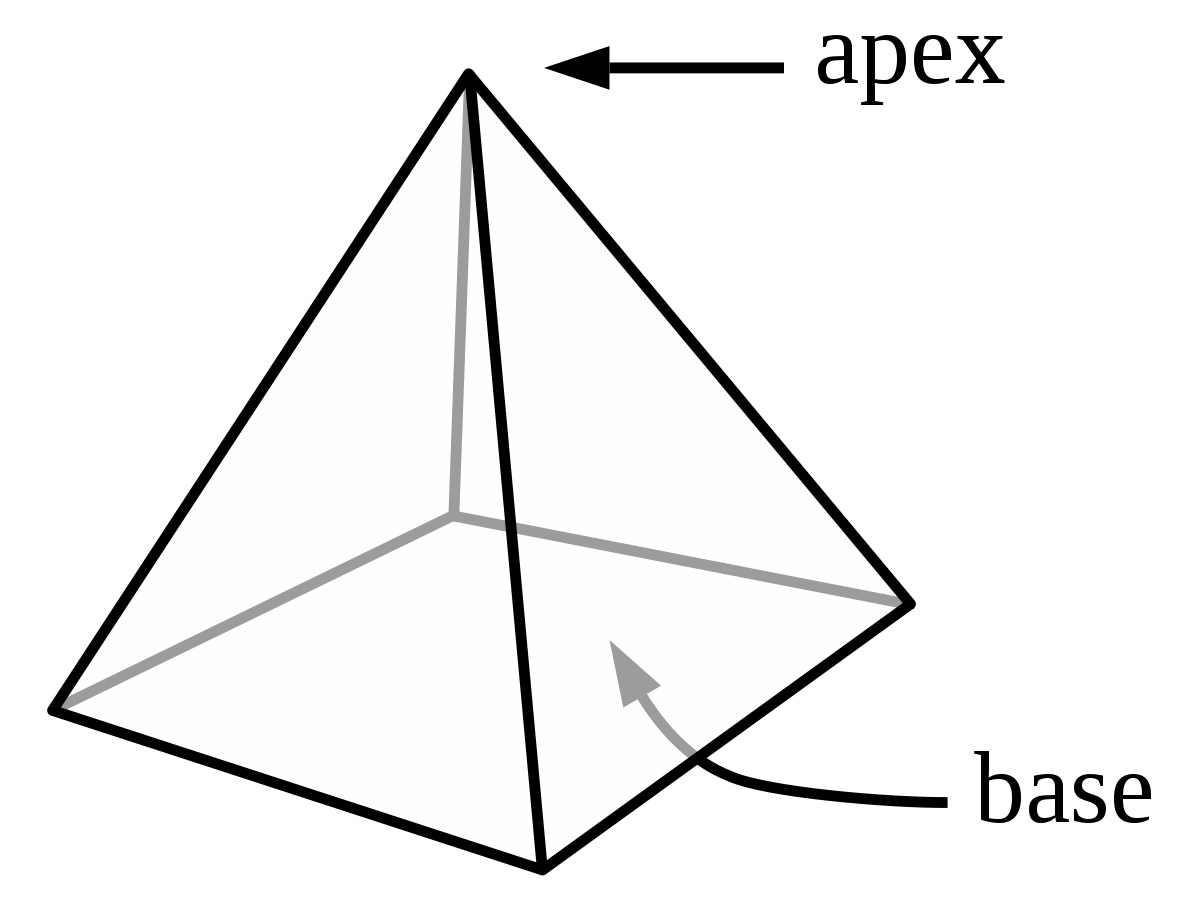# Triangular Pyramid Surface Area Calculator

Select what you want to calculate and place the values in the given input boxes to find the surface area of a regular pyramid or any other quantities related to a pyramid.

FormulaV = (l * w * h) / 3## Triangular Pyramid Surface Area Calculator

The surface area of triangular pyramid calculator handles all pyramid related calculations like a pro. It doesn’t matter if you want to find the surface area, volume, base area, base width, or base length. It offers a one-stop-shop for all calculations of a pyramid.

## What is a pyramid?

A Pyramid is a solid object having a polygon base, triangular sides that meet at the top. A Pyramid is a three-dimensional structure and a polyhedron.## Formula

The pyramid area calculator automatically utilizes the relevant formula and calculates the area, volume, base, and other terms accordingly.

It composes the required formula in the run time after gathering the values and that is why it is safe to say that it is also a surface area of a triangular pyramid formula calculator.

Triangular Pyramid
Area of the base (A) = ½ * a * s
Surface Area of Pyramid = A + ((3/2) sl)
Volume of Pyramid = (1/6) abh
Where,
a= apothem length
s= side length
sl= slant height of a pyramid
abh area of base * height

In case you don’t want to get stuck in equations, pyramid volume calculator can ease your calculations of pyramid.

Square based pyramid
Area of the base (A) [s²]
Surface Area of square Pyramid = [s² + 2sl]
Volume of Square Pyramid = [(1/3)b²h ]

The above equation can be used to calculate the square pyramid volume.
Where,
s= side length
sl= slant height
b= base
h= height

Pentagonal
Area of Base : [(5/2)as]
Surface Area of Pyramid : [ (5/2)as + (5/2)sl ]
Volume of Pyramid : [ (5/6)abh]
Where,
As = area of side length
sl= slant height
abh area of base * height

Hexagonal based pyramid
Area of Base: [(6/2) as]
Surface Area of Pyramid: [3as + 3sl]
Volume of Pyramid: [abh]
Where,
As = area of side length
sl= slant height
abh area of base * height

A pyramid is a polyhedron with one face as a base, a polygon, and all the other faces triangles meeting at a common polygon vertex as the apex. It is a structure where the upper surfaces are triangular and converge on one point.

## Surface area of a triangular pyramid calculator

Wondering how to find the surface area of a pyramid? Let’s find it step by step in this section.

### Find the area, surface area and volume of a triangular pyramid with the given apothem length 2, side 3, height 4 and the slant height 5.

Step 1: Find the area of the base.
Area of the base (A) = ½ * a * s = 0.5 * 2 * 3 = 3.

Step 2: Find the surface area of pyramid.
Surface Area of Pyramid = A + ((3/2) sl) = 3 + ((3/2) * 3 * 5) = 3 + (1.5 * 15) = 3 + 22.5 = 25.5.

Step 3: Find the volume of pyramid.
Volume of Pyramid = (1/6) abh = (1/6) * 2 * 3 * 4 = 0.17 * 24 = 4.08.

### Find the area, surface area and volume of a right square (right rectangular) pyramid with the given side length 3, height 4 and the slant height 5.

Step 1: Find the area of the base. (Right square pyramid calc: find a)
Area of the base (A) [s²] = 32 = 9
Step 2: Find the surface area of pyramid.
Surface Area of Pyramid = [s² + 2sl] = 32 +2*5= 9+10 = 9 +2*5*3 =9+30 = 39

Step 3: Find the volume of pyramid. (Right rectangular pyramid calc: find v)
Volume of Pyramid = [(1/3) b²h] = [(1/3)*92 *4] = (1/3)*9*4 = 36/3 = 12

Step 4: Find the lateral area of pyramid. (Right rectangular pyramid calc: find a_l)

Lateral area of rectangular pyramid:
A_L=l√((w/2)^2+h^2 )+w√((l/2)^2+h^2 )
A_L=3√((3/2)^2+4^2 )+3√((3/2)^2+4^2 ) A_L=3√(73/4)+3√(73/4)
AL = 12.82 + 12.82 = 25.64

### Find the area, surface area and volume of a Pentagonal pyramid with the given side apothem length 2, side 3, Slant height 4 and height 5.

Step 1: Find the area of the base.
Area of Base : [(5/2)as] = [ (5/2)2*3]= 5/2*6= 2.5*6= 15

Find the surface area of pyramid.
Surface Area of Pyramid : [ (5/2)as + (5/2)sl ]= 15+(2.5*3*4) = 15+ (2.5*12) =15+30 = 45

Find the volume of pyramid.
Volume of Pyramid : [ (5/6)abh] = (5/6) 2*3*5=  (5/6) * 30 = 0.833 *30 = 25 \

### Find the area, surface area and volume of a Hexagonal pyramid with the given side apothem length 2, side 3, Slant height 4 and height 5.

Step 1: Find the area of the base. Area of Base:  [(6/2) as] = [(6/2) 2*3= (6/2) *6 = 3*6 = 18

Find the surface area of a pyramid.
Surface Area of Pyramid:  [3as + 3sl] = [(3*2*3) + (3*3*4)] = 18+36 = 54

Find the volume of pyramid.
Volume of Pyramid:  [abh] = 2*3*5 = 30

## FAQs

### How to find the surface area of a square pyramid?

The surface area of squared pyramid can be found using the below equation:

A = a (a + √ (a2 + 4h2))

Where a represents the side length, and h is the height of the pyramid.

### How do you find the total surface area of a pyramid?

The total surface area of pyramid can be calculated using the below formula:

T.S.A = (1/2)pl + B

Where l is the slant height, B is the base area, and p represents the perimeter of the base.